Math Calculators, Lessons and Formulas

It is time to solve your math problem

mathportal.org
• Geometry
• Circles
• Triangles and Circles

# Triangles and Circles

ans:
syntax error
C
DEL
ANS
±
(
)
÷
×
7
8
9
4
5
6
+
1
2
3
=
0
.
auto next question
calculator
•  Question 1: 1 pts Each of the two triangles is isosceles, $\vartriangle ABC$ and $\vartriangle ACD.$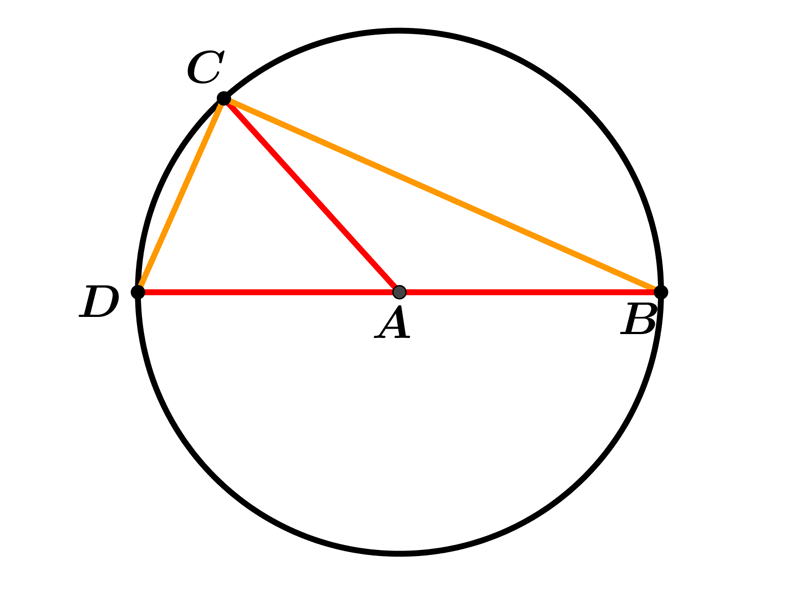•  Question 2: 1 pts Find the radius of the circle in this $5, 12, 13$ right triangle.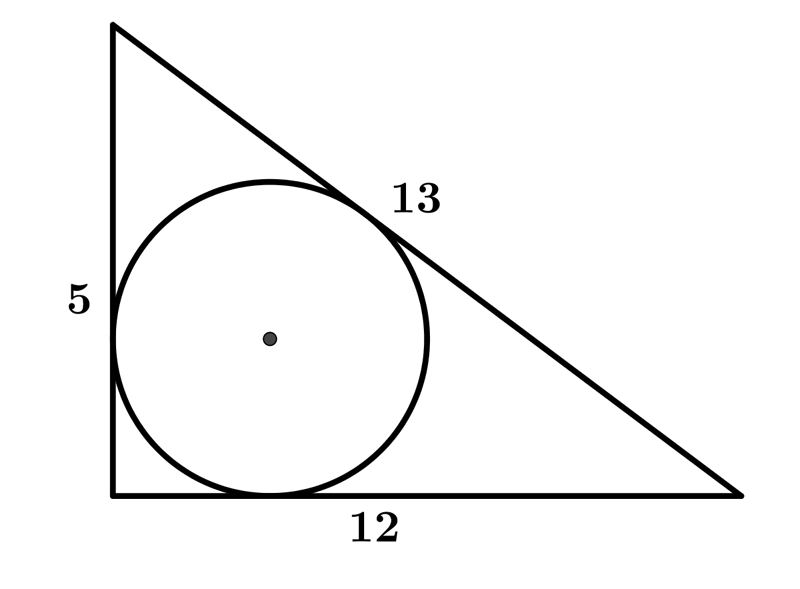Radius $=$
•  Question 3: 1 pts Find the perimeter of the shaded area shown on the picture.($\vartriangle ABC$ is an equilateral triangle.)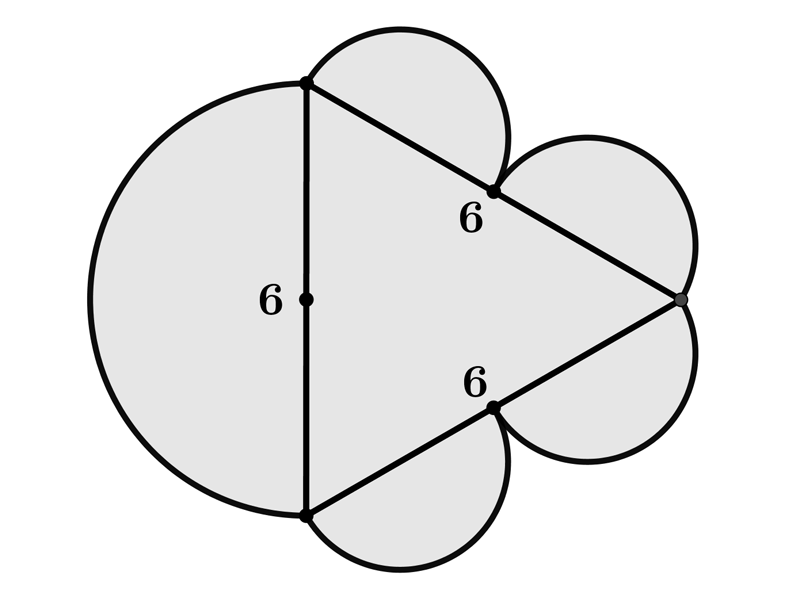$P=6\pi$ $P=12\pi$ $P=18\pi$ $P=9\pi$
•  Question 4: 1 pts Find the perimeter of the shaded area shown on the picture.($\vartriangle ABC$ is an equilateral triangle.)$P=5\pi$ $P=10\pi$ $P=15\pi$ $P=20\pi$
•  Question 5: 2 pts An equilateral triangle is inscribed in a circle. If the radius of the circle is $3\sqrt{3}cm$, which expression can be used to find the length of the side $a$ of the triangle?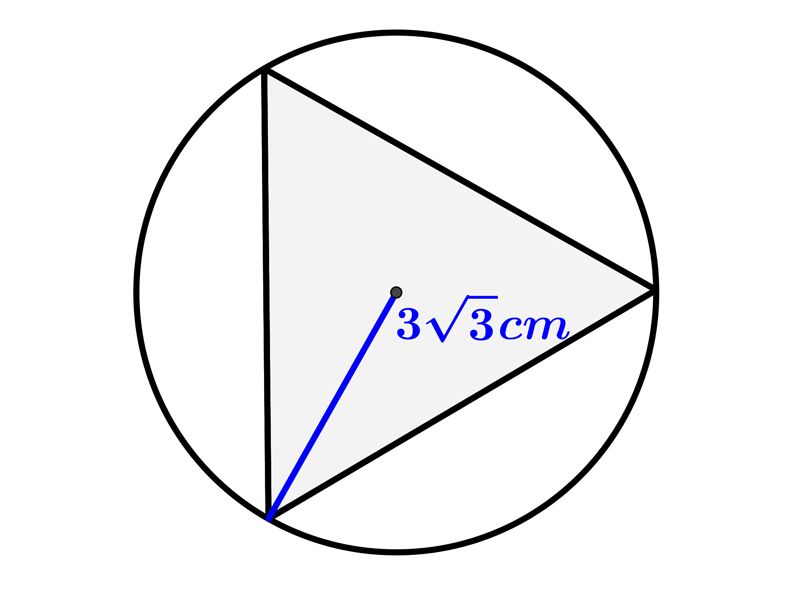$a=\dfrac{3\cdot 3}{3}cm$ $a=\dfrac{3\cdot 3\sqrt{3}}{\sqrt{3}}cm$ $a=\dfrac{3\cdot 3\sqrt{6}}{\sqrt{3}}cm$ $a=\dfrac{3\cdot 3\sqrt{3}}{\sqrt{6}}cm$
•  Question 6: 2 pts What is the area of a circle which is inscribed in a equilateral triangle of side $7cm$?
 $A=\dfrac{51}{15}\pi cm^{2}$ $A=\dfrac{48}{13}\pi cm^{2}$ $A=\dfrac{49}{12}cm^{2}$ $A=\dfrac{49}{12}\pi cm^{2}$
•  Question 7: 2 pts Find the area of the right triangle shown on the picture.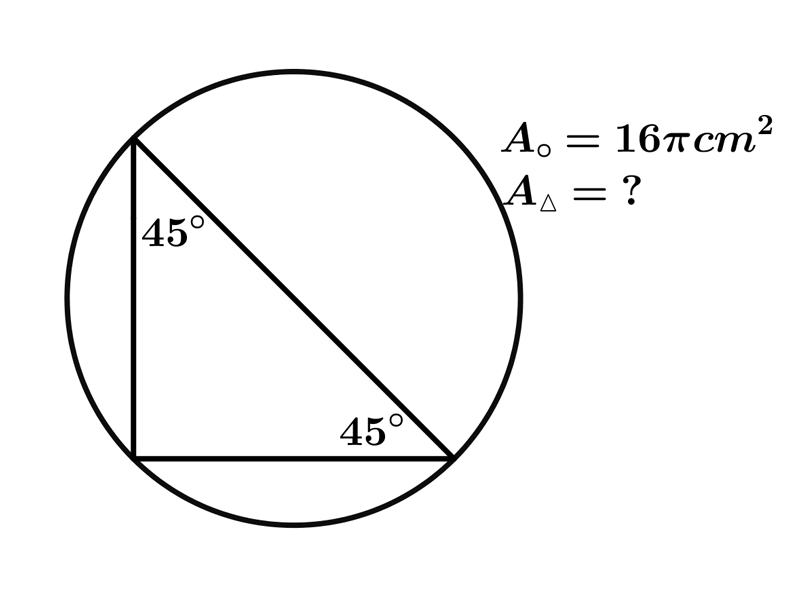$A=$
•  Question 8: 2 pts Find the area of the shaded figure shown on the picture.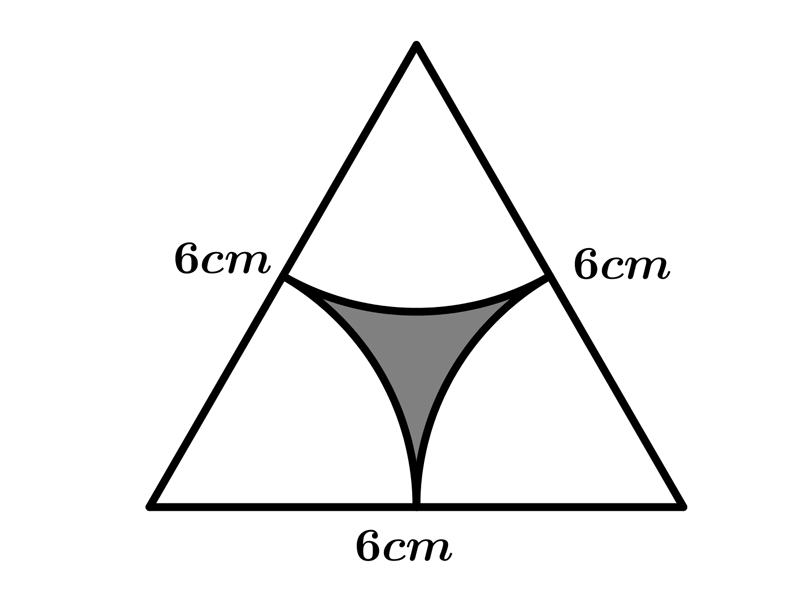$A=$
•  Question 9: 3 pts An equilateral triangle is inscribed in circle. Find the length of the longer arc $BC$ if the length of side of the triangle is $6cm.$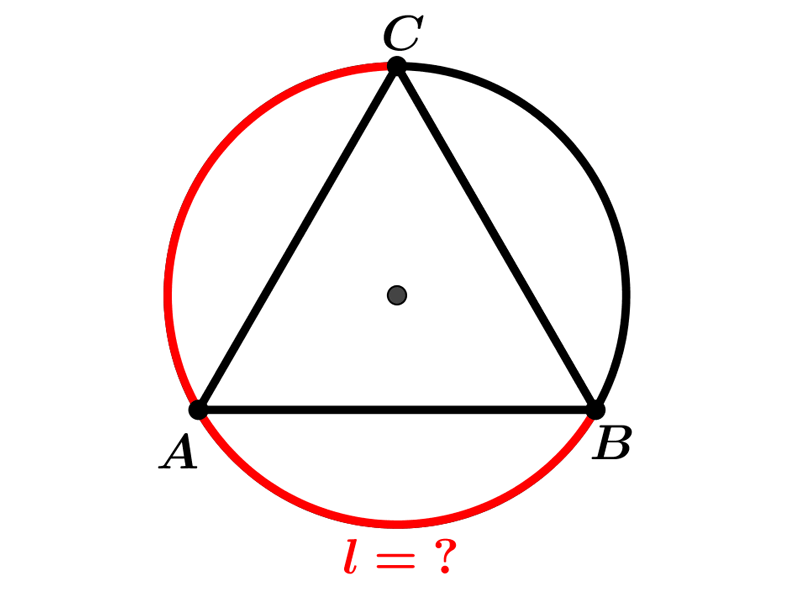$\dfrac{8\sqrt{3}}{3}\pi cm$ $\dfrac{6\sqrt{3}}{5}\pi cm$ $\dfrac{2\sqrt{3}}{3}\pi cm$ $\dfrac{3\sqrt{3}}{2}\pi cm$
•  Question 10: 1 pts Area of triangle $=\dfrac{1}{2}\cdot a\cdot r +\dfrac{1}{2}\cdot b\cdot r+\dfrac{1}{2}\cdot c\cdot r=\dfrac{(a+b+c)}{2}\cdot r$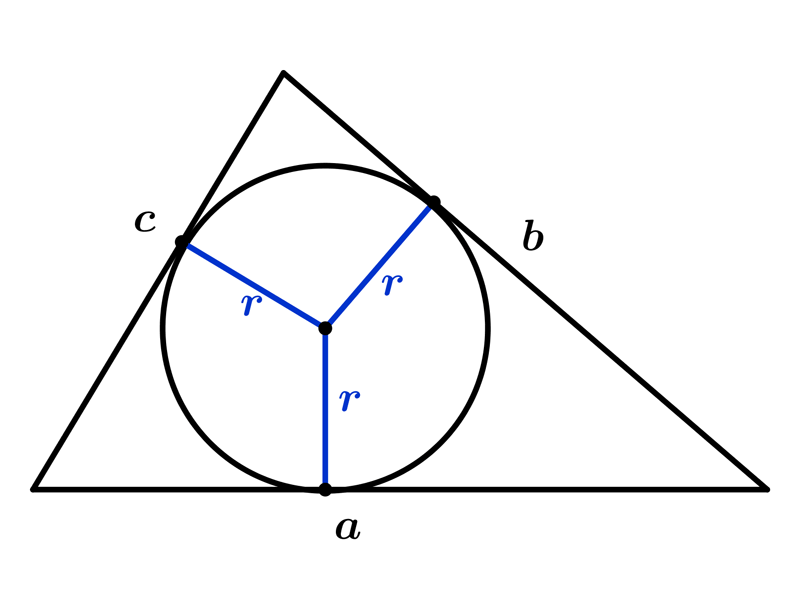•  Question 11: 3 pts $AB$ is a diameter of the circle, $AC = 6 cm$ and $BC = 8 cm.$Find the area of the shaded regio.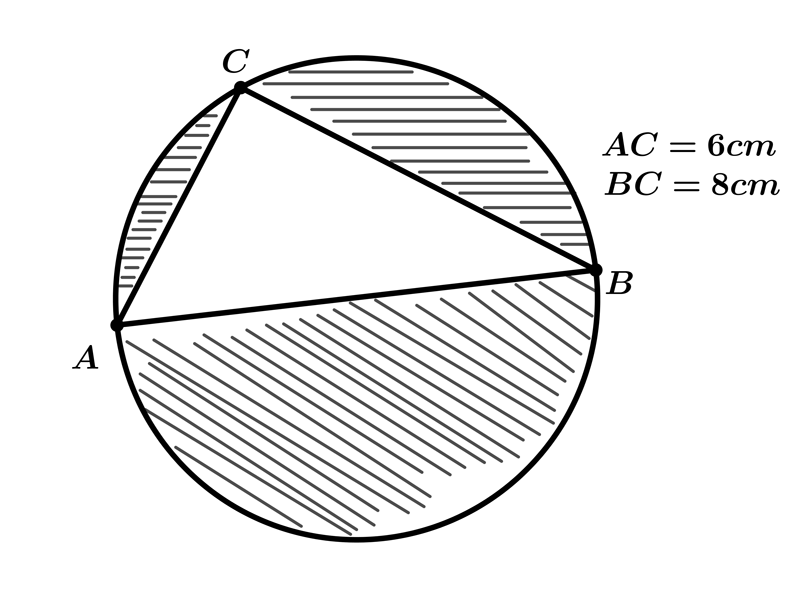$A=(25\pi-16) cm^{2}$ $A=(36\pi-25) cm^{2}$ $A=(25\pi-24) cm^{2}$ $A=(24\pi-25) cm^{2}$
•  Question 12: 3 pts In the given pictures, arcs have been drawn with radii $14 cm$ each and with centres $P, Q$ and $R.$ Find the area of the shaded region.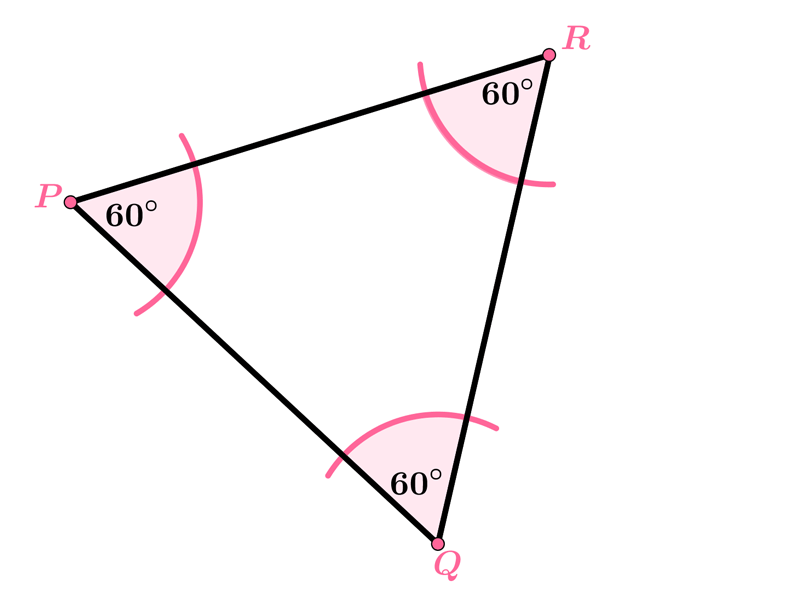$A=98\pi cm^{2}$ $A=89\pi cm^{2}$ $A=969\pi cm^{2}$ $A=196\pi cm^{2}$